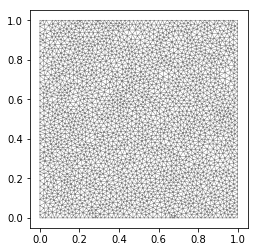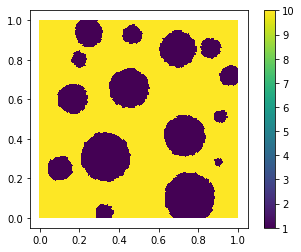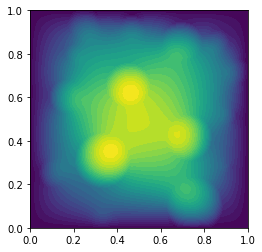## Random fields¶

In what follows, we step forward to the generation of a (input) random field $g\in L^P(\Omega,\Sigma, \mathbb{P}; \mathcal{X})$ with realisation in a Banach space $\mathcal{X}=\mathcal{X}(D)$ defined on some Lipschitz domain $D\subset\mathbb{R}^2$ for some $1<P< \infty$. For example we have $\mathcal{X} = L^\infty(D)$ for the diffusion field $g=a$ or $\mathcal{X} = H^{-1}(D)$ resp. $\mathcal{X} = L^2(D)$ for the case of right hand side $g=f$.

We will discuss two examples of random fields:

#### Implicit random field¶

As an example we present a simple random cookie field, that models composite material.

#### Explicit parametric representation¶

Here we state representation by means of Karhunen–Loève expansion (KLE): \begin{align} \kappa(x,\omega) = \kappa(x, \xi(\omega)) = \kappa_0(x) + \sum\limits_{m=1}^\infty \sqrt{\lambda_m}\kappa_m(x)\xi_m(\omega) \end{align}

##### remark¶

KLE based representation and its truncated version are one of the basis motivation for stochastic Galerkin methods based on generalized polynomial chaos expansions (gPCE), which extends Wiener's polynomial chaos expansion (w.r.t. to underlying Gaussian random variables).

### A table full of cookies¶

In the following we generate random fields by means of rejecting. This applies e.g. for coefficients describing random composite material. Here we model a matrix composite material with variable but fixed number of non-overlapping circular inclusions with random radii and positions also known as a random cookie material.

At first we load necessary packages.

In :
from dolfin import *
from random import uniform
import mshr
import numpy as np

import matplotlib as mpl
mpl.rcParams['lines.linewidth'] = 0.5

%matplotlib inline


First we generate random radii and positions uniquely describing the circular inclusions.

In :
def generate_non_overlapping_data(no_holes, size_D = [(0.,0.),(1.,1.)]):
""""
Generate radii and midpoint data for [no_holes] number of circular subdomains
that do not overlap within a given distance dist_tol = 0.001.

The midpoint lies within the region of interest specified by size_D.

@param no_holes: number of circular subdomains
@param size_D  : speficies the rectangular domain D

@returns radii and midpoint data as lists
"""
# compute noHoles configurations of voids not intersecting
# presetted range of radii of the circular subdomains

# data for subdomain circles that not overlap...
radii, m_x, m_y = [], [], []
# by minimal distance of
dist_tol = 0.005

success = 0
while success < no_holes:
# generate a sample candidate
cx_new  = uniform(size_D, size_D)
cy_new  = uniform(size_D, size_D)

# check if sample intersects with previous circular data
for rad, cx, cy in zip(radii, m_x, m_y):
distM = np.sqrt((cx_new - cx) ** 2 + (cy_new - cy) ** 2)
if rad_new + rad + dist_tol > distM:
break

m_x.append(cx_new)
m_y.append(cy_new)
success += 1

return radii, m_x, m_y


Next the above code is used to generate a realisation of the underlying random cookie field.

In :
def composite_material(no, N):
"""
Returns a realisation of a diffusion coefficient that is piecewise constant with
circular inclusions, may represent composite material.
@param no: number of holes
@param N : discretisation
:return: Fenics function and underlying mesh
"""

coeff1 = 1.
coeff2 = 10.

# define 2D geometry
size_D = [(0., 0.), (1., 1.)]
domain = mshr.Rectangle(Point(size_D, size_D), Point(size_D, size_D))

# get realisation of cookie data
radii, m_x, m_y  = generate_non_overlapping_data(no)

# set subdomains for an adapted mesh
for k, data in enumerate(zip(radii, m_x, m_y)):
r, x, y = data
circ = mshr.Circle(Point(x, y), r)
domain.set_subdomain(k+1, circ)

# generate mesh
mesh = mshr.generate_mesh(domain, N)

# a subdomain class representing a cookie used for definition of the FE function field
class circle(SubDomain):
def __init__(self, r, mx, my):
self._r = r
self._mx = mx
self._my = my
def inside(self, x, on_boundary):
return pow(x - self._mx, 2) + pow(x - self._my, 2) - pow(self._r, 2) <= dolfin.DOLFIN_EPS
circles = [ circle(r,mx,my) for r,mx,my in zip(radii, m_x, m_y)]

# creation of discrete space of piecewise constant functions w.r.t. the mesh
DG0 = FunctionSpace(mesh, "DG", 0)
# create the output field
field = Function(DG0)
# data array associated for field
data  = np.array([coeff2]*DG0.dim())

# relation between d.o.fs and topological entities (cell midpoints)...
dofs = DG0.dofmap().dofs()
dofs_x = DG0.tabulate_dof_coordinates().reshape((-1, mesh.geometry().dim()))
dofVertexMap = zip(dofs, dofs_x)

# ... used to set the FE function field
for dof, x in dofVertexMap:
for circle in circles:
if circle.inside(x, False):
data[dof] = coeff1
break
field.vector()[:] = data

return field, mesh


Now we generate a mesh and a cookie field for $15$ cookies.

In :
field, mesh = composite_material(15, 40)


We plot the underlying mesh to observe the grain circles.

In :
plot(mesh)

Out:
[<matplotlib.lines.Line2D at 0x7fa251ee9e10>,
<matplotlib.lines.Line2D at 0x7fa251ee9fd0>]Now plot the field (based on matplotlib new mesh data). The field takes the value $1.0$ inside the circle and $10.0$ in the outer media.

In :
plt_field = plot(field)    # fenics.plot returns a matplotlib object
mpl.pyplot.colorbar(plt_field)

Out:
<matplotlib.colorbar.Colorbar at 0x7fa251e6fe50># Exercise I¶

Numerically solve the diffusion problem with homogeneous Dirichlet b.c. using the cookie field as diffusion coefficient and plot the discrete solution.

In :
fe_type = "Lagrange"
fe_degree = 1
V = FunctionSpace(mesh, fe_type, fe_degree)

# Define boundary condition
u0 = Constant(0.0)
bc = DirichletBC(V, u0, 'on_boundary')

# Define variational problem
u = TrialFunction(V)
v = TestFunction(V)
kappa = field
f = Constant(1) # Expression("1", degree=3)
L = f*v*dx

# Compute solution
u = Function(V)
solve(a == L, u, bc)

plot(u)

Out:
<matplotlib.tri.tricontour.TriContourSet at 0x7fa24d3e7c50>End of part II. Next: random fields Part 2

© Robert Gruhlke, Manuel Marschall, Phillip Trunschke, 2018-2019